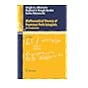Normal view

# Mathematical theory of Feynman path integrals : an introduction / Sergio A. Albeverio, Raphael J. Høegh-Krohn, Sonia Mazzucchi.

Material type:TextSeries: Lecture notes in mathematics (Springer-Verlag) ; 523.Publication details: ©2008. Edition: 2nd corr. and enl. edDescription: 1 online resource (x, 175 pages)Content type: text Media type: computer Carrier type: online resourceISBN: 9783540769569; 3540769560; 3540769544; 9783540769545Genre/Form: Electronic books. Additional physical formats: Print version:: Mathematical theory of Feynman path integrals.DDC classification: 515.43 LOC classification: QC174.17.F45 | A42 2008ebQA3 | .L28 no. 523 2008ebOnline resources: Click here to access online
Contents:
Preface to the second edition -- Preface to the first edition -- 1.Introduction -- 2.The Fresnel Integral of Functions on a Separable Real Hilbert Spa -- 3.The Feynman Path Integral in Potential Scattering -- 4.The Fresnel Integral Relative to a Non-singular Quadratic Form -- 5.Feynman Path Integrals for the Anharmonic Oscillator -- 6.Expectations with Respect to the Ground State of the Harmonic Oscillator -- 7.Expectations with Respect to the Gibbs State of the Harmonic Oscillator -- 8.The Invariant Quasi-free States -- 9.The Feynman Hystory Integral for the Relativistic Quantum Boson Field -- 10.Some Recent Developments -- 10.1.The infinite dimensional oscillatory integral -- 10.2.Feynman path integrals for polynomially growing potentials -- 10.3.The semiclassical expansio -- 10.4.Alternative approaches to Feynman path integrals -- 10.4.1.Analytic continuation -- 10.4.2.White noise calculus -- 10.5.Recent applications -- 10.5.1.The Schroedinger equation with magnetic fields -- 10.5.2.The Schroedinger equation with time dependent potentials -- 10.5.3 .hase space Feynman path integrals -- 10.5.4.The stochastic Schroedinger equation -- 10.5.5.The Chern-Simons functional integral -- References of the first edition -- References of the second edition -- Analytic index -- List of Notations.
Summary: Feynman path integrals, suggested heuristically by Feynman in the 40s, have become the basis of much of contemporary physics, from non-relativistic quantum mechanics to quantum fields, including gauge fields, gravitation, cosmology. Recently ideas based on Feynman path integrals have also played an important role in areas of mathematics like low-dimensional topology and differential geometry, algebraic geometry, infinite-dimensional analysis and geometry, and number theory. The 2nd edition of LNM 523 is based on the two first authors' mathematical approach of this theory presented in its 1st edition in 1976. To take care of the many developments since then, an entire new chapter on the current forefront of research has been added. Except for this new chapter and the correction of a few misprints, the basic material and presentation of the first edition has been maintained. At the end of each chapter the reader will also find notes with further bibliographical information.
Tags from this library: No tags from this library for this title.
Star ratingsAverage rating: 0.0 (0 votes)
Holdings
Item type Current library Collection Call number Status Date due Barcode Item holdseBook e-Library

Electronic Book@IST

EBook Available
Total holds: 0

Includes bibliographical references and index.

Print version record.

Preface to the second edition -- Preface to the first edition -- 1.Introduction -- 2.The Fresnel Integral of Functions on a Separable Real Hilbert Spa -- 3.The Feynman Path Integral in Potential Scattering -- 4.The Fresnel Integral Relative to a Non-singular Quadratic Form -- 5.Feynman Path Integrals for the Anharmonic Oscillator -- 6.Expectations with Respect to the Ground State of the Harmonic Oscillator -- 7.Expectations with Respect to the Gibbs State of the Harmonic Oscillator -- 8.The Invariant Quasi-free States -- 9.The Feynman Hystory Integral for the Relativistic Quantum Boson Field -- 10.Some Recent Developments -- 10.1.The infinite dimensional oscillatory integral -- 10.2.Feynman path integrals for polynomially growing potentials -- 10.3.The semiclassical expansio -- 10.4.Alternative approaches to Feynman path integrals -- 10.4.1.Analytic continuation -- 10.4.2.White noise calculus -- 10.5.Recent applications -- 10.5.1.The Schroedinger equation with magnetic fields -- 10.5.2.The Schroedinger equation with time dependent potentials -- 10.5.3 .hase space Feynman path integrals -- 10.5.4.The stochastic Schroedinger equation -- 10.5.5.The Chern-Simons functional integral -- References of the first edition -- References of the second edition -- Analytic index -- List of Notations.

Feynman path integrals, suggested heuristically by Feynman in the 40s, have become the basis of much of contemporary physics, from non-relativistic quantum mechanics to quantum fields, including gauge fields, gravitation, cosmology. Recently ideas based on Feynman path integrals have also played an important role in areas of mathematics like low-dimensional topology and differential geometry, algebraic geometry, infinite-dimensional analysis and geometry, and number theory. The 2nd edition of LNM 523 is based on the two first authors' mathematical approach of this theory presented in its 1st edition in 1976. To take care of the many developments since then, an entire new chapter on the current forefront of research has been added. Except for this new chapter and the correction of a few misprints, the basic material and presentation of the first edition has been maintained. At the end of each chapter the reader will also find notes with further bibliographical information.

## Other editions of this workMathematical theory of Feynman path integrals / by Albeverio, Sergio. ©1976Mathematical theory of Feynman path integrals / by Albeverio, Sergio. ©1976Mathematical theory of Feynman path integrals / by Albeverio, Sergio. ©1976
Share